Number CountsNext: Redshift Distribution Up: The Faint Galaxy Previous: The Local Galaxy

# Number Counts

The simplest way to study galaxies is to count how many there are as a function of apparent magnitude per unit area (usually deg) on the sky. Number counts are usually denoted by N(m). It was thought that N(m) could be used to constrain cosmological models [\protect\astronciteHubble and Tolman1935]. For example, in an Euclidean universe uniformly populated by galaxies with the same intrinsic luminosity, the count slope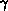=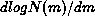should be equal to 0.6. It was soon realized that galaxy evolution could have at least as much of an effect on N(m) as the geometry of the Universe. Also, number counts have a built-in weak point which makes their interpretation difficult: galaxies with a wide range of intrinsic luminosities are included in each apparent magnitude bin. Number counts are the results of integrating over intrinsic luminosity and distance.

Early faint galaxy counts showed a marked number excess over standard models incorporating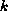-corrections but neglecting evolutionary effects, and an overall blueing trend. Shanks et al. shanks84 obtained band rgalaxy number counts and colour distributions down to B23. At b= 23, they observed three times as many galaxies as predicted by a q= 0.02 no-evolution model, but rcounts were much closer to model counts. In the range 19.5 < r< 20.5, galaxy colors appeared to be shifted towards the blue with respect to the data at brighter magnitudes, and with respect to the prediction of the no-evolution model. Koo koo86 presented UBVI counts and color distributions of10000 galaxies to B24. He found that U counts rose rapidly with magnitude with= 0.68. Galaxies fainter than B20 also had progressively more of an ultraviolet excess eventually exceeding that of Galactic subdwarfs. The fraction of field galaxies intrinsically bluer than BV = 0.7 increased to74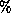by z0.4 from the local value of0.4. Tyson tyson88 conducted a very deep multicolor imaging survey of 25000 galaxies that revealed strong evidence for color and luminosity evolution. Galaxy counts at b= 25 were a factor 515 above no-evolution models. The mean galaxy colours approached bR = 0, RI = 0.8 at the faint limit compared to bR2.0 at brighter magnitudes (R = 21).

More recent galaxy number counts confirmed the trends seen in earlier works. Metcalfe et al. met91 determined B and R galaxy counts for 21 < B < 25 and 19 < R < 23.5. They also found that B counts were systematically higher than no-evolution model predictions. There was a factor of three excess in the magnitude range 24.5 < B < 25. The status of galaxy counts as of 1993 was neatly presented in Figure 1 of Lilly lilly93 reproduced in Figure. Figureshows galaxy counts in B, I and K plotted against various models. The lower solid curve and the dashed curve are no-evolution models with q= 0.5 and q= 0 respectively. The dot-dashed curve is a non-standard cosmological model with a non-zero cosmological constant (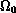= 0.1,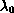= 0.9). The upper solid curve represents an evolving model in which the luminosities of Irr galaxies have been arbitrarily increased by a factor of 41. Figureclearly shows that the excess is more pronounced in B than in K. The K counts are almost fitted with a non-standard cosmology. The B counts cannot even be fitted with the extreme evolving model shown here. The color dependence of the excess is interpreted as increased star formation in faint galaxies. Different bandpasses look at different stellar populations within galaxies. The B bandpass looks at the young, star-forming component of galaxies whereas K looks at more long-lived, quiescent stellar populations. K band luminosities are therefore more representative of the masses of galaxies and are less affected by galaxy evolution.

Cowie et al. cow91 obtained redshifts and K-band magnitudes for 22 galaxies with B magnitudes down to 24. At B = 2324, 3080of the galaxies were small, blue galaxies with median M, redshift and BK color of21.3 (L = 0.01 L), 0.24 and 3.4 respectively. To explain such a large fraction of low-luminosity galaxies, a steep rise in the Schechter function faint magnitudes was required. This population of low-luminosity objects contained as much K-band light as the normal galaxy population, which meant they contained as much baryonic matter as the normal galaxy population. K-band counts over the range 10 < K < 23 [\protect\astronciteGardner et al.1993] showed a change in slope at K17 from= 0.67 to= 0.26. At K17, the median BK color as a function of K magnitude turned over, and galaxies fainter than K = 17 rapidly became bluer. Models with non-zerodid not appear to fit the counts: they overpredicted the faint end counts if evolution was added, and they underpredicted the bright end counts with no evolution. Gardner et al. expected their K magnitude-limited sample to be dominated by Kelliptical (Kis the K magnitude corresponding to the Schechter luminosity L) and Sa galaxies. Since a passively evolving Kelliptical galaxy reaches K = 17 at z1, their data indicated that the population of field galaxies had undergone an evolutionary changes by z1 and that the colors were bluer than expected.

The Hubble Space Telescope (HST) has added a new dimension to number counts. As discussed in section, it is now possible with HST to determine galaxy number counts as a function of galaxy types and identify what population is responsible for the excess of faint galaxies.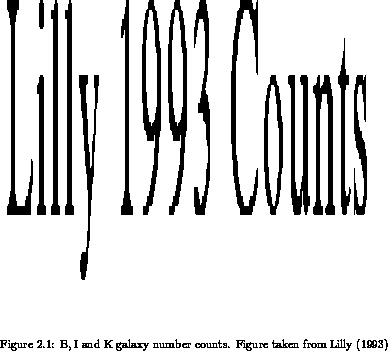Next: Redshift Distribution Up: The Faint Galaxy Previous: The Local Galaxy

Luc Simard
Mon Sep 2 12:37:40 PDT 1996